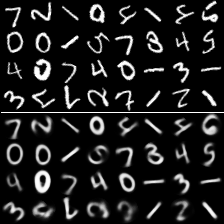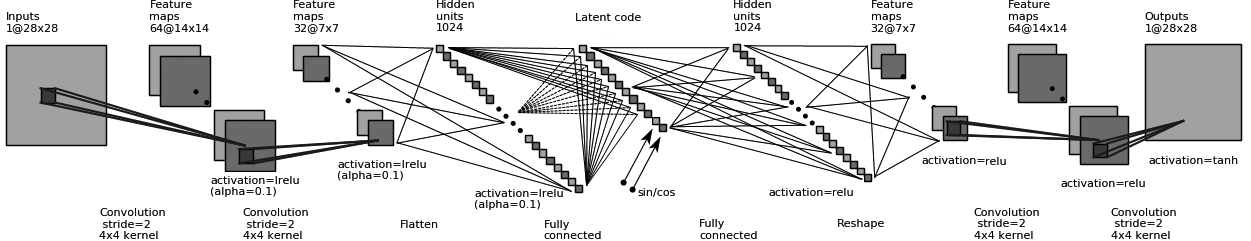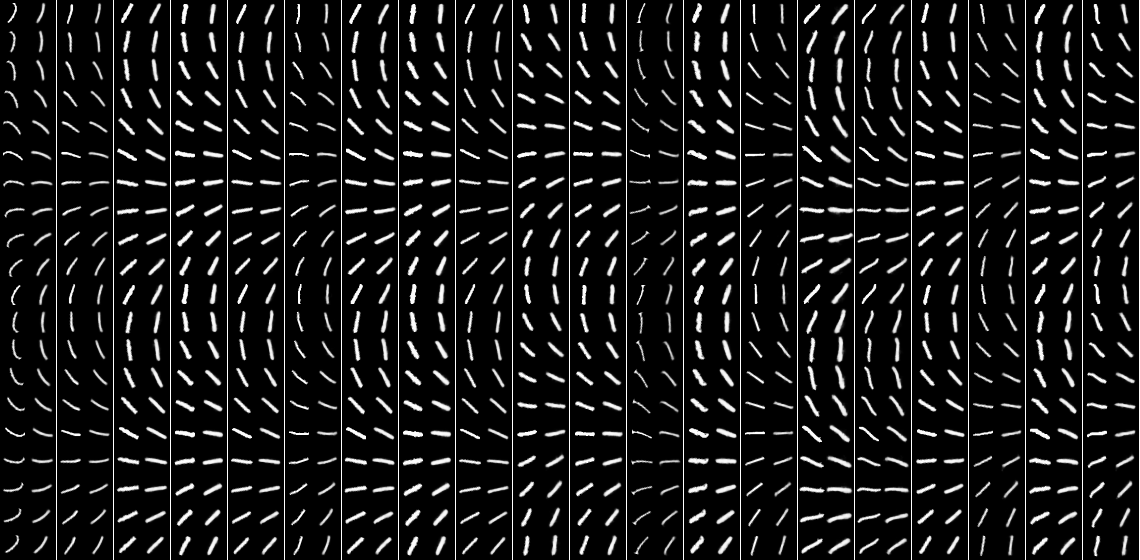# Can Deep Networks Learn Invariants?

### Let's look at Artificial General Intelligence (AGI) and how it should be able to learn to recognize new classes of objects from as few examples as possible.

· AI Zone · Analysis
Save
6.31K Views

Artificial General Intelligence (AGI) should be able to see. In particular, it should be able to recognize objects and to learn to recognize new classes of objects from as few examples as possible.

This means that it should generalize. How would that work?

Imagine that we have a pattern x that can be transformed by some transformation T, and transformed patterns T(x|w) for all values of the transformation parameters w should be identified with the original pattern. The transformation is not known. That is, either the transformation itself or invariant recognition model should be learned.

Deep neural networks (DNNs) are very successful in computer vision, but can they learn invariants or generalize outside the region of training sets, or can they only interpolate and memorize? Our team at the SingularityNET AI research lab has been experimenting with these possibilities:

## Recognition

### Experimental Setup

Common transformations, to which recognition models should be invariant, are spatial transformations. Invariance to shifts is usually hard-coded by the use of convolutional neural networks (CNNs). Rotation-invariant CNNs are also sometimes used, but not too popular. The usual technique to achieve invariant recognition is to extend training sets with spatially transformed versions of original images.

Will this help to recognize objects in new poses?

Ideally, a machine learning system should be able to extrapolate beyond the range of parameter values seen in the training set. For example, if we train a recognition model on all MNIST digits rotated within the range [–45ᵒ,45ᵒ], it should be able to recognize these digits rotated by, e.g., 90ᵒ.

We started with a simpler task. We extended the MNIST training set (with removed "6" and "9" digits) with all digits except ‘3’ and ‘4’ rotated within the whole range of angles, while digits "3" and "4" rotated within [–45ᵒ,45ᵒ]. The basic yet instructive experiment is to see whether it will be possible or not for DNN models to recognize digits "3" and "4" rotated by the angles outside the range [–45ᵒ,45ᵒ] without an explicit introduction of rotation-invariant capabilities.

Note: There are current models capable of learning spatial transformations (e.g. Spatial Transformers), in particular, to make recognition models invariant to them. Although such a solution can be quite practical and more general than hard-coded invariance to concrete transformations, it still supposes that the class of transformations and their appropriate parameterization is known. We are interested in capabilities of DNNs to achieve invariance to a priori unknown transformations.

An example of a more relevant work is “Non-Local Estimation of Manifold Structure,” which considers precisely the task of extrapolation beyond the area of training set and transferring this extrapolation (e.g. rotation manifold structure) to novel image classes. Unfortunately, the authors study only "slight rotations," which application to novel images already yields non-perfect results. Thus, we consider general-purpose DNN models.

### CNNs

We took basic CNN networks for first experiments. Our baseline network contained two convolutional layers and two dense layers with softmax as output layer (code is available here). We considered the following accuracy tests:

• Accuracy on the whole test set with the rotation angles from the same range as was used during training: Ptest=0.989.
• Accuracy on digits 3 and 4 (images from the test set) with the rotation angles from [45ᵒ, 315ᵒ]: Pout=0.212.
• Accuracy on digits 3 and 4 (images from the test set) with the rotation angles from [135ᵒ, 225ᵒ]: Pinv=0.003.

It can be seen that the model poorly generalizes outside the area of the training set, and its accuracy degrades almost to zero (far below random guess) for the range of angles far outside the training set, even if it is trained on all rotation angles for other digits.

It is known that such techniques as batch normalization (BN) improve generalization capabilities of neural networks. We tried both BN and dropout and their combination. The best model achieved Ptest=0.993, Pout=0.257, Pinv=0.041. It shows higher accuracy in general, but it is still far below random guess for the invariance test.

One can assume that the network is not deep enough, and deeper features might be able to extrapolate the manifold structure better. However, in our experiments, the addition of more layers to the best model with two convolutional layers slightly improved Ptest, Pout, but also slightly decreased Pinv.

Thus, conventional CNNs fail to generalize the idea of rotation without additional means (not just to extrapolate to never seen rotation angles, but to transfer recognition capabilities for encountered angles from one class to another).

### CapsNets

Capsule networks (CapsNets) were specifically designed for capturing the part-whole relationship taking into account poses of objects and their parts. We consider capsule networks here since they don’t operate explicitly with spatial locations and transformations, but utilize a general mechanism of inference-time routing between neuronal layers, which relies on the vector output of capsules.

First, we considered CapsNet with dynamic routing. An original implementation of it based on the paper “Dynamic Routing Between Capsules” was taken from here.

For this implementation of CapsNet, we achieved Ptest=0.991, Pout=0.249, Pinv=0.009, which is not better than that of CNNs with BN (and invariance is worse).

This CapsNet model contains a decoder, which is used for regularization and can also be used to see what information is contained in the highest-level representation of patterns. An example of trained decoder output is following (input images are in the upper half, decoded images are in the lower half).CapsNet: decoded images

The reconstruction results for images in encountered earlier orientations are reasonable. However, if we look at the following results of reconstruction of digits 3 and 4 in novel orientations, they are completely incorrect and resemble other digits (since they are recognized as such).CapsNet: decoded images in novel orientations

We also studied the performance of CapsNets with EM-routing using this implementation.

The following score were achieved: Ptest=0.976, Pout=0.218, Pinv=0.090. Interestingly, this CapsNet shows the best score in the invariance test, although its accuracy on not extended test set is not too high, but it is below the level of random guess (so it didn’t generalize, but simply didn’t overfit much).

Our experiments in more detail are presented here.

## Generation

### Experimental setup

Generative models are very important in the context of AGI. In particular, an AGI system should have an imagination capable of rendering images of novel objects transformed in different ways (e.g., observed from different viewpoints, but not only). We will not go into detail here, but simply ask if generative networks are capable of learning to perform “mental rotation.”

We trained models to construct a rotated version of an input image given information about rotation angle. Since we are interested in generative models, we used autoencoders in our experiments. An encoder transforms an input image to some latent code, which is concatenated with encoded rotation angle, which is fed to directly to a decoder, and then the decoder is required to reconstruct a properly rotated image. We found that the best way to represent the input angle a to the model is by the pair of values: cos(a) and sin(a).Convolutional autoencoder architecture

Models were trained on the MNIST dataset. Original digit images from the MNIST training set were fed as input, while rotated images were required as output. For each training sample, the rotation angle was randomly chosen from [–180ᵒ, 180ᵒ] for all digits except 4 and 9, for which the range was [–45ᵒ, 45ᵒ]. It should be noted that there was no problem with confusing 6 and 9 since the rotation angle was provided to models. Then, the rotation of images from the test set was evaluated.

### Rotation Test

We conducted experiments with the autoencoder composed of the encoder consisted of two convolutional layers with kernel=4 and stride=2 (64 and 128 feature maps), intermediate dense layer with 1024 units and last dense layer with 10 units constituting the latent code, and the decoder consisted of the same number of layers and units, but with reverted connections. The whole latent code contained two variables receiving cos(a) and sin(a) in addition to 10 variables, which values were calculated by the encoder. We considered both vanilla (non-regularized) autoencoders trained with the reconstruction loss and adversarial autoencoders trained with two updates (reconstruction loss and adversarial loss). The former showed somewhat worse reconstruction results, but are more useful as generative models. Implementations can be found here.

Autoencoders were trained end-to-end to reconstruct rotated images, so we hoped that encoders would learn useful latent representations.

Trained models were able to reconstruct new images of known digits rotated by angles within the ranges of the training set almost perfectly (left columns contain expected results of reconstruction — not the input images, while right columns contain actual results of reconstruction):Reconstruction results

However, the reconstruction error for angles outside the range [–45ᵒ, 45ᵒ] for digit ‘9’ appeared to be large, and for digit "4" — much larger. Peaks on 0ᵒ and ±90ᵒ should be ignored, because they are connected to bilinear blurring of images for all angles differ from 0ᵒ and ±90ᵒ.Reconstruction error for different rotation angles (normalized to [-1,1] range) and different digits

Consider the following example of reconstructed (rotated) images of "4" and "9" for different angles.Reconstruction results

Although in some cases, the result of rotation of "4" to large angles is not too bad, but it is far from perfect and tends to resemble other digits. The situation for "9" is somewhat better, because the network learned to draw ‘6’ in all orientations. It seems that the model successfully extrapolated the connection between the latent code of "6" and "9" beyond the range of angles presented in the training set, but it didn’t generalize the procedure of rotation. It can also be seen that the model transforms "9" to "0," "1" or "7" in some cases.

Thus, the model failed not just to extrapolate rotation to novel angles, but also to apply its acquired capability to rotate some digits to other digits. Thus, we conclude that the model mostly memorizes how should look different digits rotated by different angles, although some generalization takes place.

### Shift Test

We conducted a similar experiment, but for shifts instead of rotation. One could expect that there should be no problem with learning how to reconstruct a shifted version of the image since a convolutional decoder is used. However, the result is the same as for rotations.Reconstruction with shifts

This result is easily understandable. Latent code neurons have individual connections to each neuron of the layer, which is interpreted as a set of feature maps, for which (transposed) convolution is then applied. Apparently, weights of connections leading to each area of these feature maps are learned independently.

• If the model learned to activate proper features to draw a digit at one place, it will not help the model to draw the same digit at another place because corresponding connections will not be trained.
• If the decoder is fed with a digit latent code and a novel shift, it will activate neurons of the feature map in the proper place, but they will correspond to a mixture of features of digits with similar latent codes, for which the model knows how to draw them in this position.

Thus, the model doesn’t shift the image of a digit to a novel position but assembles it from images (fused on the level of features) of other digits. Of course, if we try to reconstruct a digit with shift, which was not present in the training set at all, the model will fail to render anything with this shift.

Experiments with autoencoders are described here in more detail.

As we have seen, neural networks fail to learn (generalize/extrapolate) invariants in discriminative settings or transformations in generative settings, although they don’t just memorize patterns and show some form of generalization.

Although traditional neural networks are very powerful models, aiming at the creation of AGI will likely require the creation of new neural models capable of true generalization, or the connection of DNN nodes to a sort of symbolic induction nodes, which will help to overcome restrictions of each other.

The results of these experiments have helped fuel new possibilities for neural networks capable of producing AGI. We’ll introduce some of our early work regarding these initiatives in a future post.

Topics:
artificial intelligence, agi, neural networks, deep learning, machine learning, analysis, invariants, deep networks

Published at DZone with permission of Alexey Potapov.

Opinions expressed by DZone contributors are their own.